# Op Amp Configurations Inverting Amplifier Non Inverting Amplifier

• Slides: 23Op Amp Configurations Inverting Amplifier Non Inverting Amplifier Summing Amplifier Operational Amplifier CHAPTER 8 © Electronics Open loop mode Two main characteristics vo = Aod ( v 2 – v 1) v 1 = v 2 to create Aod = No current going into the op-amp If you don’t remember the formulas, remember these two characteristics and perform nodal analysis EECE 1312Chapter 2 Semiconductor Materials and Diodes © Electronics EECE 1312Classification of Materials Classification according to the way materials react to the current when a voltage is applied across them: l Insulators Ø Materials with very high resistance - current can’t flow Ø mica, rubber l Conductors Ø Materials with very low resistance – current can flow easily Ø copper, aluminum l Semiconductors Ø Neither good conductors nor insulators (silicon, germanium) Ø Can be controlled to either insulators by increasing their resistance or conductors by decreasing their resistance © Electronics EECE 1312Semiconductor Materials and Properties ● An atom is composed of a nucleus, which contains positively charged protons and neutral neutrons, and negatively charged electrons that orbit the nucleus. ● Electrons in the outermost shell are called valence electrons. © Electronics EECE 1312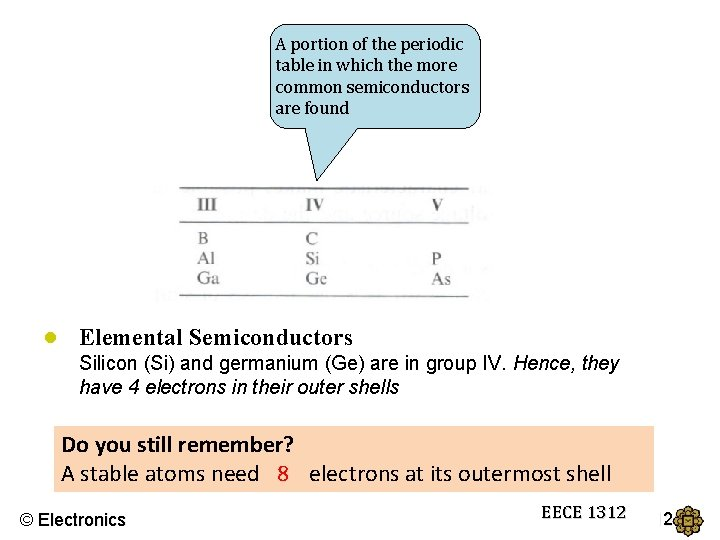A portion of the periodic table in which the more common semiconductors are found ● Elemental Semiconductors Silicon (Si) and germanium (Ge) are in group IV. Hence, they have 4 electrons in their outer shells Do you still remember? A stable atoms need 8? electrons at its outermost shell © Electronics EECE 1312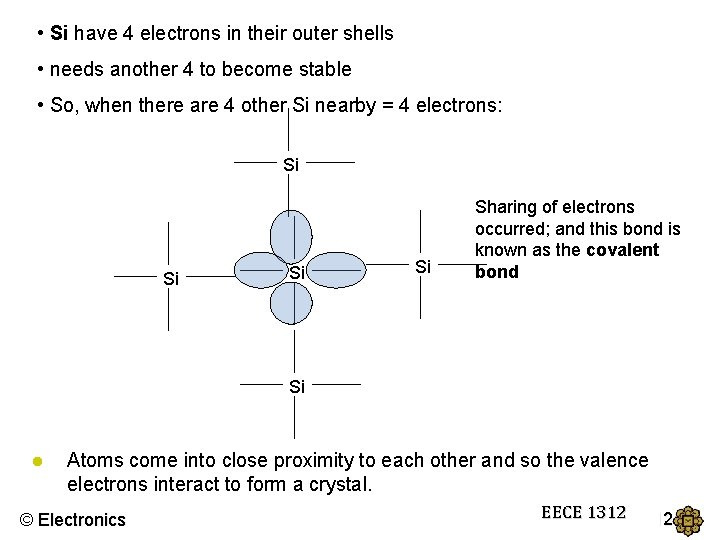• Si have 4 electrons in their outer shells • needs another 4 to become stable • So, when there are 4 other Si nearby = 4 electrons: Si Si Sharing of electrons occurred; and this bond is known as the covalent bond Si ● Atoms come into close proximity to each other and so the valence electrons interact to form a crystal. © Electronics EECE 1312BANDGAP ENERGY, Eg • Now, in order to break the covalent bond, a valence electron must gain enough energy to become free electrons. • The minimum energy required is known as the bandgap energy, Eg © Electronics EECE 1312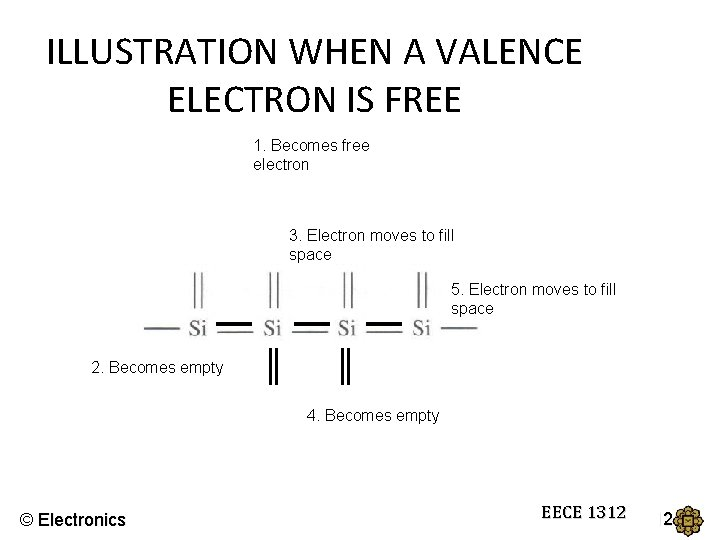ILLUSTRATION WHEN A VALENCE ELECTRON IS FREE 1. Becomes free electron 3. Electron moves to fill space 5. Electron moves to fill space 2. Becomes empty 4. Becomes empty © Electronics EECE 1312Intrinsic Semiconductor ● Intrinsic Semiconductor Ø A single-crystal semiconductor material with no other types of atoms within the crystal. Ø The densities of electrons and holes are equal. Ø The notation ni is used as intrinsic carrier concentration for the concentration of the free electrons as well as that of the hole: B = a coefficient related to the specific semiconductor material Eg = the bandgap energy (e. V) T = the temperature (Kelvin) remember that K = °C + 273. 15 k = Boltzmann’s constant (86 x 10 -6 e. V/K) © Electronics EECE 1312Intrinsic Semiconductors Cont. The energy difference between the minimum conduction band energy and the maximum valance band energy is called bandgap energy. Semiconductor Constants Material Bandgap Energy (e. V) B (cm-3 K-3/2) Silicon (Si) 1. 1 5. 23 × 1015 Germanium (Ge) 0. 66 1. 66 × 1015 Gallium Arsenide (Ga. As) 1. 4 2. 10 × 1014 © Electronics EECE 1312● The values of B and Eg for several semiconductor materials: EXAMPLE 1 Calculate the intrinsic carrier concentration in silicon at T = 300 K. © Electronics EECE 1312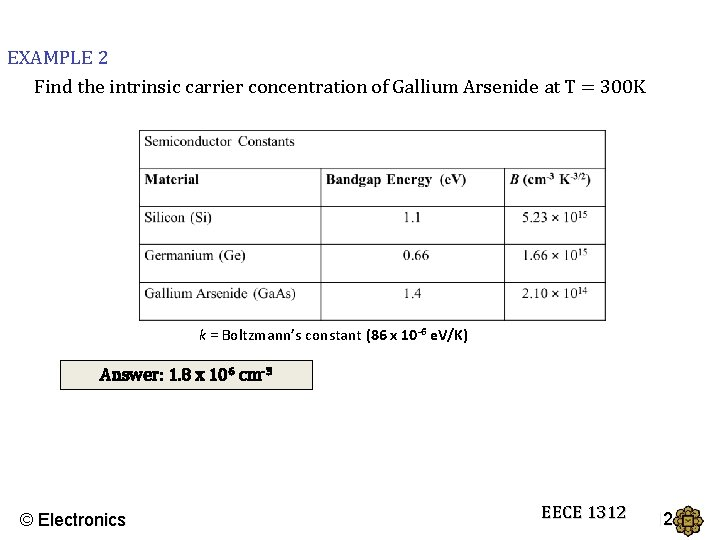EXAMPLE 2 Find the intrinsic carrier concentration of Gallium Arsenide at T = 300 K k = Boltzmann’s constant (86 x 10 -6 e. V/K) Answer: 1. 8 x 106 cm-3 © Electronics EECE 1312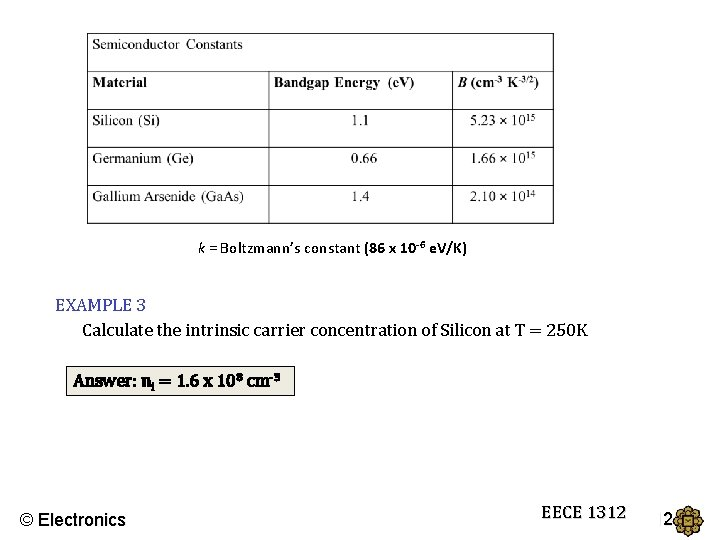k = Boltzmann’s constant (86 x 10 -6 e. V/K) EXAMPLE 3 Calculate the intrinsic carrier concentration of Silicon at T = 250 K Answer: ni = 1. 6 x 108 cm-3 © Electronics EECE 1312Extrinsic Semiconductor • Since intrinsic concentration, ni is very small, so, very small current is possible • So, to increase the number of carriers, impurities are added to the Silicon/Germanium. • The impurities will be from Group V and Group III © Electronics EECE 1312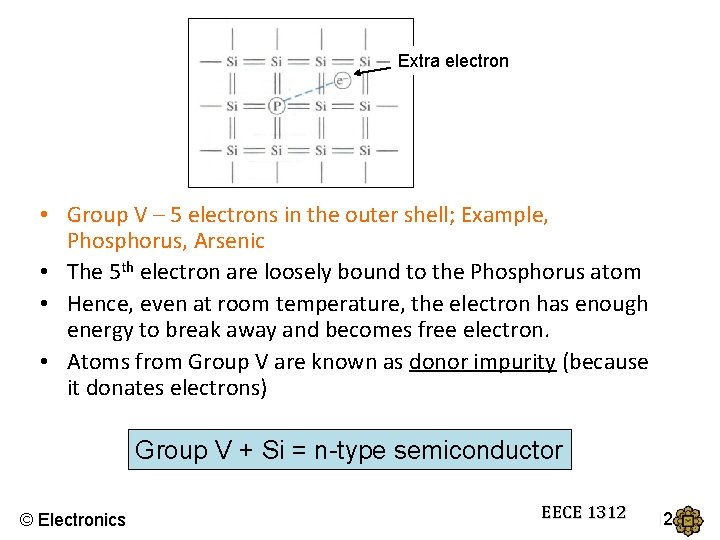Extra electron • Group V – 5 electrons in the outer shell; Example, Phosphorus, Arsenic • The 5 th electron are loosely bound to the Phosphorus atom • Hence, even at room temperature, the electron has enough energy to break away and becomes free electron. • Atoms from Group V are known as donor impurity (because it donates electrons) Group V + Si = n-type semiconductor © Electronics EECE 1312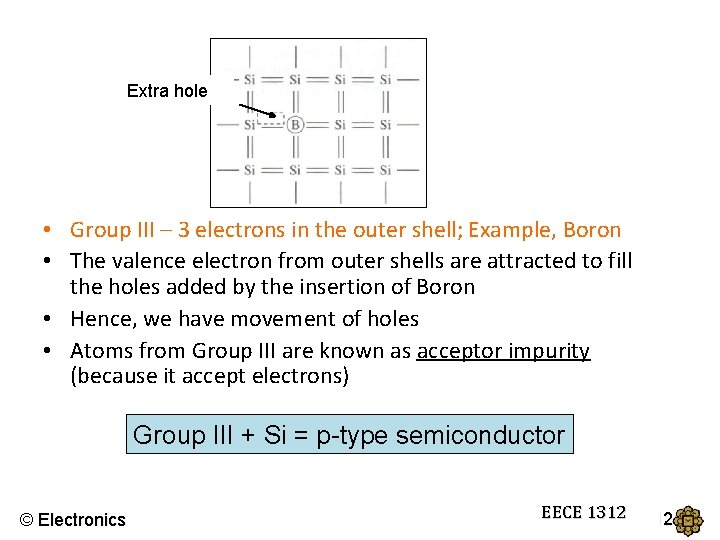Extra hole • Group III – 3 electrons in the outer shell; Example, Boron • The valence electron from outer shells are attracted to fill the holes added by the insertion of Boron • Hence, we have movement of holes • Atoms from Group III are known as acceptor impurity (because it accept electrons) Group III + Si = p-type semiconductor © Electronics EECE 1312The Fermi level shifts due to doping Fermi level © Electronics EECE 1312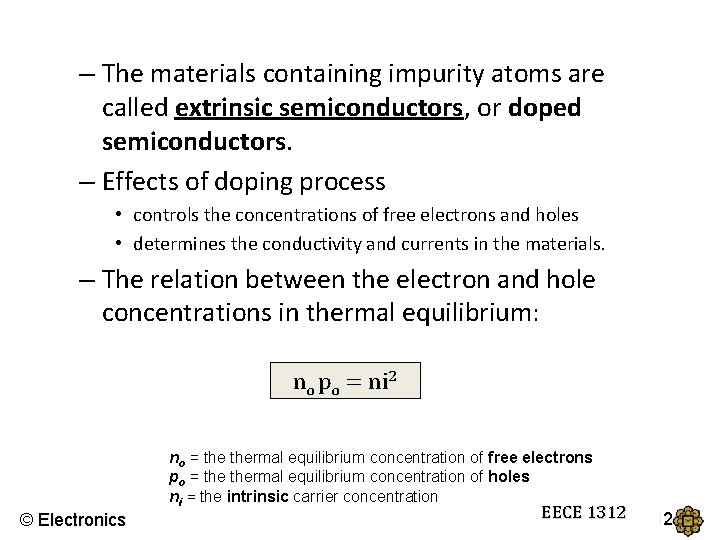– The materials containing impurity atoms are called extrinsic semiconductors, or doped semiconductors. – Effects of doping process • controls the concentrations of free electrons and holes • determines the conductivity and currents in the materials. – The relation between the electron and hole concentrations in thermal equilibrium: no po = ni 2 no = thermal equilibrium concentration of free electrons po = thermal equilibrium concentration of holes ni = the intrinsic carrier concentration © Electronics EECE 1312For N-type – electrons are the majority carriers In thermal equilibrium, generally in the n-type semiconductor the donor atom concentration Nd is much higher than the intrinsic carrier concentration, called majority charge carrier. And the hole concentration in the n-type semiconductor is called minority charge carrier. © Electronics EECE 1312For P-type – holes are the majority carriers Similarly in thermal equilibrium concentration the majority charge carrier free hole in the p-type semiconductor is And the minority charge carrier electron concentration in the p-type semiconductor is © Electronics EECE 1312Example 1 Calculate thermal equilibrium electron and hole concentrations. Consider silicon at T = 300 K doped with phosphorous at a concentration of Nd = 1016 cm-3 and ni = 1. 5 x 1010 cm-3. © Electronics EECE 1312Example 2 Calculate the majority and minority carrier concentrations in silicon at T = 300 K if a) b) Na = 1017 cm-3 Nd = 5 x 1015 cm-3 1. Calculate ni 2. For part (a) – it is p-type 3. For part (b) – it is n-type Answer: a) majority = 1017 cm-3 minority = 2. 25 x 103 cm-3 b) majority = 5 x 1015 cm-3, minority 4. 5 x 104 cm-3 © Electronics EECE 1312k = Boltzmann’s constant (86 x 10 -6 e. V/K) Example 3 A silicon is doped with 5 x 1016 arsenic atoms a) Is the material n-type or p-type? b) Calculate the electrons and holes concentration of the doped silicon at T=300 K Answer: a) n-type b) no = 5 x 1016 cm-3 and po = 4. 5 x 103 cm 3 © Electronics EECE 1312